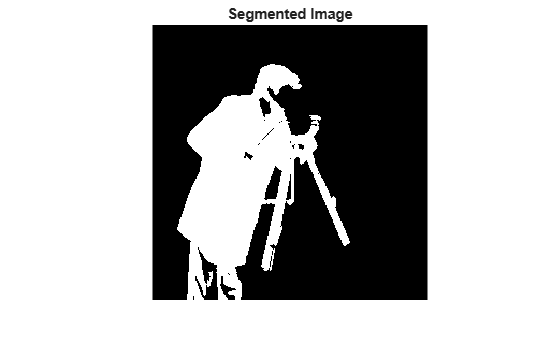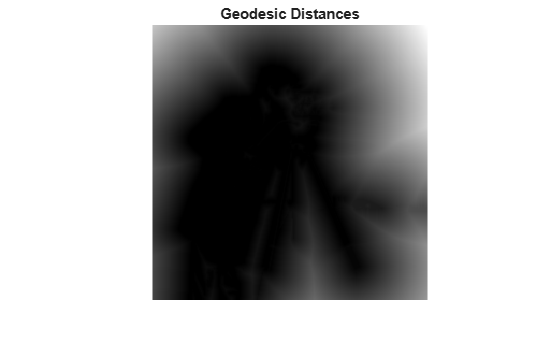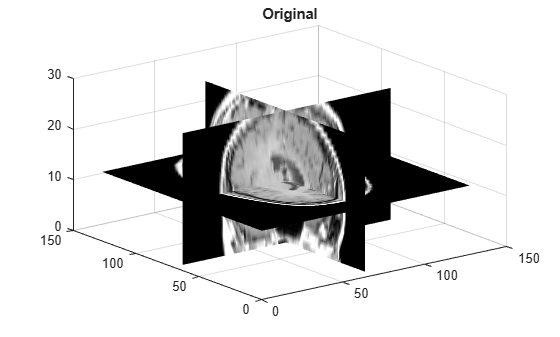imsegfmm

Binary image segmentation using fast marching method

Description

example

BW = imsegfmm(W,mask,thresh) returns a segmented image BW, which is computed using the fast marching method. The array W specifies weights for each pixel. mask is a logical array that specifies seed locations. thresh specifies the threshold level.

BW = imsegfmm(W,C,R,thresh) returns a segmented image, with seed locations specified by the vectors C and R, which contain column and row indices. C and R must contain values which are valid pixel indices in W.

BW = imsegfmm(W,C,R,P,thresh) returns a segmented image, with seed locations specified by the vectors C, R, and P, which contain column, row, and plane indices. C, R, and P must contain values which are valid pixel indices in W.

[BW,D] = imsegfmm(___) returns the normalized geodesic distance map D computed using the fast marching method. BW is a thresholded version of D, where all the pixels that have normalized geodesic distance values less than thresh are considered foreground pixels and set to true. D can be thresholded at different levels to obtain different segmentation results.

Examples

collapse all

This example shows how to segment an object in an image using Fast Marching Method based on differences in grayscale intensity as compared to the seed locations.

imshow(I)
title('Original Image')Create mask and specify seed location. You can also use roipoly to create the mask interactively.

Compute the weight array based on grayscale intensity differences.

W = graydiffweight(I, mask, 'GrayDifferenceCutoff', 25);

Segment the image using the weights.

thresh = 0.01;
[BW, D] = imsegfmm(W, mask, thresh);
figure
imshow(BW)
title('Segmented Image')You can threshold the geodesic distance matrix D using different thresholds to get different segmentation results.

figure
imshow(D)
title('Geodesic Distances')This example segments the brain from MRI data of the human head.

V = squeeze(D);

Visualize the data.

sizeO = size(V);
figure
slice(double(V),sizeO(2)/2,sizeO(1)/2,sizeO(3)/2);
colormap("gray")
title("Original")Set the seed locations.

seedR = 75;
seedC = 60;
seedP = 10;

Compute weights based on grayscale intensity differences.

W = graydiffweight(V,seedC,seedR,seedP,"GrayDifferenceCutoff",25);

Segment the image using the weights.

thresh = 0.002;
BW = imsegfmm(W,seedC,seedR,seedP,thresh);

Visualize the segmented image using an isosurface.

figure
p = patch(isosurface(double(BW)));
p.FaceColor = "red";
p.EdgeColor = "none";
daspect([1 1 27/64]);
camlight
lighting phongInput Arguments

collapse all

Weight array, specified as a non-negative numeric array. You can compute the weight array by using the graydiffweight or gradientweight functions. Large values in W identify the foreground (object) and small values identify the background.

Data Types: single | double | uint8 | int8 | int16 | uint16 | int32 | uint32

Seed locations mask, specified as a logical array of the same size as W. Locations where mask is true are seed locations. If you use graydiffweight to create the weight matrix W, it is recommended that you use the same value of mask with imsegfmm that you used with graydiffweight.

Data Types: logical

Threshold level used to obtain the binary image, specified as a number in the range [0, 1]. Low values typically result in large foreground regions (logical true) in BW, and high values produce small foreground regions.

Example: 0.5

Data Types: double

Column index of reference pixels, specified as a numeric vector.

Example: [50 75 93]

Data Types: double

Row index of reference pixels, specified as a numeric vector.

Example: [48 71 89]

Data Types: double

Plane index of reference pixels, specified as a numeric vector.

Example: [2 4 7]

Data Types: double

Output Arguments

collapse all

Segmented image, returned as a logical array of the same size as W.

Data Types: logical

Normalized geodesic distance map, returned as a numeric array of the same size as W. If W is of class single, then D is of class single. Otherwise, D is of class double.

Data Types: double | single

Tips

• imsegfmm uses double-precision floating point operations for internal computations for all classes except class single. If W is of class single, imsegfmm uses single-precision floating point operations internally.

• imsegfmm sets pixels with 0 or NaN weight values to Inf in the geodesic distance image D. These pixels are part of the background (logical false) in the segmented image BW.

 Sethian, J. A. Level Set Methods and Fast Marching Methods: Evolving Interfaces in Computational Geometry, Fluid Mechanics, Computer Vision, and Materials Science, Cambridge University Press, 2nd Edition, 1999.